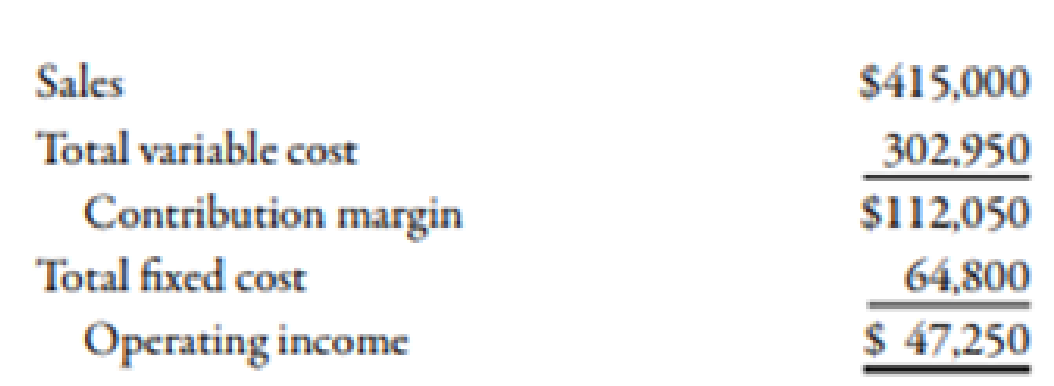Chapter 7, Problem 42E### Managerial Accounting: The Corners...

7th Edition
Maryanne M. Mowen + 2 others
ISBN: 9781337115773

#### Solutions

Chapter
Section### Managerial Accounting: The Corners...

7th Edition
Maryanne M. Mowen + 2 others
ISBN: 9781337115773
Textbook Problem
65 views

# Sales Revenue Approach, Variable Cost Ratio, Contribution Margin RatioArberg Company’s controller prepared the following budgeted income statement for the coming year:Required: 1. What is Arberg’s variable cost ratio? What is its contribution margin ratio? 2. Suppose Arberg’s actual revenues are $30,000 more than budgeted. By how much will operating income increase? Give the answer without preparing a new income statement 3. How much sales revenue must Arberg earn to break even? Prepare a contribution margin income statement to verify the accuracy of your answer. 4. What is Arberg’s expected margin of safety? 5. What is Arberg’s margin of safety if sales revenue is$380,000?

1.

To determine

Compute variable cost ratio and contribution margin ratio.

Explanation

Variable Cost Ratio:

Variable cost ratio can be evaluated by dividing the total amount of variable cost by the sales. It can also be determined by dividing the variable cost per unit by the selling price per unit.

Contribution Margin Ratio:

The sales percentage left after covering the amount of total variable cost is known as the contribution margin ratio. It is the available sales dollar percentage which is used to cover the total fixed cost.

Use the following formula to calculate the variable cost ratio:

Variable cost ratio=Total variable costTotal sales

Substitute $302,950 for total variable cost and$415,000 for total sales in the above formula.

Variable cost ratio=$302,950$415,000=0

2.

To determine

Compute the amount of increase in operating income.

3.

To determine

Calculate the sales revenue to be earned by the company at break-even. Also, prepare an income statement.

4.

To determine

Calculate the expected margin of safety.

5.

To determine

Calculate the margin of safety if the sales revenue is \$380,000.

### Still sussing out bartleby?

Check out a sample textbook solution.

See a sample solution

#### The Solution to Your Study Problems

Bartleby provides explanations to thousands of textbook problems written by our experts, many with advanced degrees!

Get Started